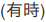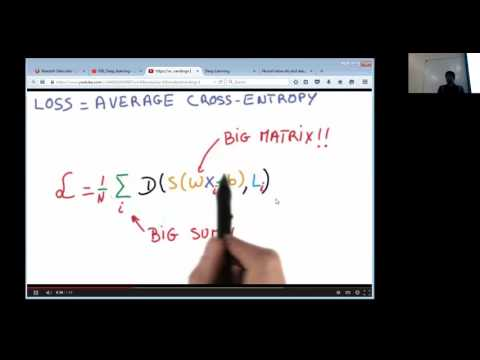# Scalable Data Science

### prepared by Paul Brouwers, Raazesh Sainudiin and Sivanand Sivaram

The html source url of this databricks notebook and its recorded Uji:# Distributed labeling of images using TensorFlow

Home work notebook for week 9.

This is essentially a tested copy of the databricks community edition notebook:

This tutorial shows how to run TensorFlow models using Spark and Databricks. At the end of this tutorial, you will be able to classify images on a Spark cluster, using a neural network.

TensorFlow is a new framework released by Google for numerical computations and neural networks. TensorFlow models can directly be embedded within pipelines to perform complex recognition tasks on datasets. This tutorial shows how to label a set of images, from a stock neural network model that was already trained.

If the classClusterTensorFlow cluster is running already then you can just attach this notebook to it and start carrying on with this tutorial.

This notebook should work on the cluster named classClusterTensorFlow on this shard (either attach your notebook to this cluster or create and attach to a cluster named classClusterTensorFlow as instructed in the companion notebook `033_SetupCluster_SparkTensorFlow`). If you want to run this script on a larger cluster, you need to follow the setup instructions in this notebook.

Let’s sky-dive into official TensorFlow website to get a view from the “stratosphere” with enough oxygen :)

### Installing TensorFlow

The TensorFlow library needs to be installed directly on the nodes of the cluster. Running the next cell installs it on your cluster if it is not there already. Running this command may take one minute or more.

``````
try:
import tensorflow as tf
except ImportError:
print "Installing TensorFlow"
import subprocess
print "TensorFlow has been installed on this cluster"

``````

TensorFlow runs as a regular Python library. The following command runs a very simple TensorFlow program.

``````
def my_function(i):
import tensorflow as tf
with tf.Session():
return tf.constant("Hello, TensorFlow!").eval()

print sc.parallelize(range(5)).map(my_function).collect()

``````

### Labeling images

We are now going to take an existing neural network model that has already been trained on a large corpus (the Inception V3 model), and we are going to apply it to images downloaded from the internet.

The code in the next cell contains some utility functions to download this model from the internet. For the purpose of this notebook it is not critical to understand what it is doing.

``````
# Imports:
import numpy as np
import tensorflow as tf
import os
from tensorflow.python.platform import gfile
import os.path
import re
import sys
import tarfile
from subprocess import Popen, PIPE, STDOUT
from six.moves import urllib
def run(cmd):
p = Popen(cmd, shell=True, stdin=PIPE, stdout=PIPE, stderr=STDOUT, close_fds=True)
from PIL import Image
import matplotlib.image as mpimg
import matplotlib.pyplot as plt

# All the constants to run this notebook.

model_dir = '/tmp/imagenet'
image_file = ""
num_top_predictions = 5

IMAGES_INDEX_URL = 'http://image-net.org/imagenet_data/urls/imagenet_fall11_urls.tgz' # ? modify this
# The number of images to process.
image_batch_size = 10
max_content = 5000L

"""Reads the index file from ImageNet (up to a limit),
and returns the content (pairs of image id, image url) grouped in small batches.
"""
from six.moves import urllib
content = urllib.request.urlopen(IMAGES_INDEX_URL)
tmpfile = "/tmp/imagenet.tgz"
with open(tmpfile, 'wb') as f:
f.write(data)
run("tar -xOzf %s > /tmp/imagenet.txt" % tmpfile)
with open("/tmp/imagenet.txt", 'r') as f:
lines = [l.split() for l in f]
input_data = [tuple(elts) for elts in lines if len(elts) == 2]
return [input_data[i:i+image_batch_size] for i in range(0,len(input_data), image_batch_size)]

Returns:
dict from integer node ID to human-readable string.
"""
label_lookup_path = os.path.join(model_dir, 'imagenet_2012_challenge_label_map_proto.pbtxt')
uid_lookup_path = os.path.join(model_dir, 'imagenet_synset_to_human_label_map.txt')
if not gfile.Exists(uid_lookup_path):
tf.logging.fatal('File does not exist %s', uid_lookup_path)
if not gfile.Exists(label_lookup_path):
tf.logging.fatal('File does not exist %s', label_lookup_path)

uid_to_human = {}
p = re.compile(r'[n\d]*[ \S,]*')
for line in proto_as_ascii_lines:
parsed_items = p.findall(line)
uid = parsed_items
human_string = parsed_items
uid_to_human[uid] = human_string

# Loads mapping from string UID to integer node ID.
node_id_to_uid = {}
for line in proto_as_ascii:
if line.startswith('  target_class:'):
target_class = int(line.split(': '))
if line.startswith('  target_class_string:'):
target_class_string = line.split(': ')
node_id_to_uid[target_class] = target_class_string[1:-2]

# Loads the final mapping of integer node ID to human-readable string
node_id_to_name = {}
for key, val in node_id_to_uid.items():
if val not in uid_to_human:
tf.logging.fatal('Failed to locate: %s', val)
name = uid_to_human[val]
node_id_to_name[key] = name

return node_id_to_name

from six.moves import urllib
dest_directory = model_dir
if not os.path.exists(dest_directory):
os.makedirs(dest_directory)
filename = DATA_URL.split('/')[-1]
filepath = os.path.join(dest_directory, filename)
if not os.path.exists(filepath):
filepath2, _ = urllib.request.urlretrieve(DATA_URL, filepath)
print("filepath2", filepath2)
statinfo = os.stat(filepath)
tarfile.open(filepath, 'r:gz').extractall(dest_directory)
else:

def display_image(url):
filename = url.split('/')[-1]
filepath = os.path.join(model_dir, filename)
urllib.request.urlretrieve(url, filepath)
image = os.path.join(model_dir, filename)
image_png = image.replace('.jpg','.png')
Image.open(image).save(image_png,'PNG')
plt.imshow(img)
display()

``````

``````
model_path = os.path.join(model_dir, 'classify_image_graph_def.pb')
with gfile.FastGFile(model_path, 'rb') as f:

``````

We are now going to download some image URLs from the ImageNet project. ImageNet is a large collection of images from the internet that is commonly used as a benchmark in image recognition tasks.

``````
num_images = sum([len(batch) for batch in batched_data])
print "There are %d images grouped in %d batches" % (num_images, len(batched_data))

``````

The labeling process can now start. We are going to use Spark to schedule the labeling of the images across our cluster, using TensorFlow.

The neural network model is quite large (250MB), so we will share it across the cluster using Spark’s broadcasting mechanism: once it is loaded onto a machine, it will not be loaded again.

``````

``````

We can now write the code that runs on each executor. It is split into two methods:

• the function `run_image` that takes a TensorFlow session already containing the graph of computations as well as a URL. This function fetches the image from the internet, passes it to the neural network and returns the list of predictions for this method
• the function `run_image_batch` that takes a set or URLs returns predictions for each of them. This is the function called by Spark. For efficiency reasons, it loads the graph of computations once before running the whole batch of images sequentially.
``````
# Functions: run_image and run_image_batch
def run_image(sess, img_id, img_url, node_lookup):
"""Fetches an image from the web and uses the trained neural network to infer the topics of this image."""
from six.moves import urllib
from urllib2 import HTTPError
try:
softmax_tensor = sess.graph.get_tensor_by_name('softmax:0')
predictions = sess.run(softmax_tensor,
{'DecodeJpeg/contents:0': image_data})
except HTTPError:
return (img_id, img_url, None)
except:
# a) The data returned may be invalid JPEG
return (img_id, img_url, None)
predictions = np.squeeze(predictions)
top_k = predictions.argsort()[-num_top_predictions:][::-1]
scores = []
for node_id in top_k:
if node_id not in node_lookup:
human_string = ''
else:
human_string = node_lookup[node_id]
score = predictions[node_id]
scores.append((human_string, score))
return (img_id, img_url, scores)

def apply_batch(batch):
with tf.Graph().as_default() as g:
graph_def = tf.GraphDef()
graph_def.ParseFromString(model_data_bc.value)
tf.import_graph_def(graph_def, name='')
with tf.Session() as sess:
labelled = [run_image(sess, img_id, img_url, node_lookup_bc.value) for (img_id, img_url) in batch]
return [tup for tup in labelled if tup is not None]

``````

Let us see how the function `run_image` performs with a portrait of Grace Hopper, one of the most famous women in Computer Sciences:

``````
display_image(url)

``````

Here is the inference results we get for this image, which is quite accurate:

``````
with tf.Graph().as_default() as g:
graph_def = tf.GraphDef()
graph_def.ParseFromString(model_data)
tf.import_graph_def(graph_def, name='')
with tf.Session() as sess:
res = run_image(sess, None, url, node_lookup)[-1]
for (keyword, weight) in res:
print '{:.8}: {}'.format(str(weight), keyword)

``````

This code is now going to be run on the dataset using Spark:

Two Runs with just 8 nodes of size 6GB RAM per node.

``````
urls = sc.parallelize(batched_data, numSlices=len(batched_data))
labelled_images = urls.flatMap(apply_batch)
local_labelled_images = labelled_images.collect()

``````
``````
urls = sc.parallelize(batched_data, numSlices=len(batched_data))
labelled_images = urls.flatMap(apply_batch)
local_labelled_images = labelled_images.collect()

``````

Now let us just change the cluster settings (`Clusters -> Configure ... ` and use dropdown menu and change number of nodes to 4).

Run with just 4 nodes.

``````
urls = sc.parallelize(batched_data, numSlices=len(batched_data))
labelled_images = urls.flatMap(apply_batch)
local_labelled_images = labelled_images.collect()

``````
``````
urls = sc.parallelize(batched_data, numSlices=len(batched_data))
labelled_images = urls.flatMap(apply_batch)
local_labelled_images = labelled_images.collect()

``````

Let us have a look at one of the images we just classified:

``````
(_, url, tags) = local_labelled_images
display_image(url)

``````
``````
tags

``````

You Try changing one of the images by changing ‘50’ below to some number between 0 and 109.

``````
len(local_labelled_images)

``````
``````
(_, url, tags) = local_labelled_images
display_image(url)

``````
``````
tags

``````

This is the end of this tutorial. You can clone this tutorial and modify it to suit your needs. Enjoy!

# Scalable Object Recognition in the Cloud for a Swarm of Robots

### Venue: Room KF7, Kirkwood Village, University of Canterbury, Kirkwood Avenue, Christchurch, New Zealand

#### by Raazesh Sainudiin done as a student project (near live)

• Google used GPUs to train the model we saw earlier
• Use the best tool for the job but use Spark to integrate predictions from multiple images streaming in from a swarm of robots, for example.

#### Can we load the pre-trained model into flying drones to let them scout out which trees have fruits ready for picking?

Let’s get some apple images (and try other fruits too) to test model identification capabilities from a google search on images for:

• “fruit trees”, “unripe fruit trees”, etc.
• finding the url of the image and feeding it to the pre-trained model,
• and seeing how well the pre-trained model does.
``````
url = "http://static1.squarespace.com/static/548b6971e4b0af3bfe38cd6f/t/56a7d76c42f5526d030146c8/1462916503492/Fruit-Tree-Apple-Tree.jpg"
display_image(url)

``````
``````
with tf.Graph().as_default() as g:
graph_def = tf.GraphDef()
graph_def.ParseFromString(model_data)
tf.import_graph_def(graph_def, name='')
with tf.Session() as sess:
res = run_image(sess, None, url, node_lookup)[-1]
for (keyword, weight) in res:
print '{:.8}: {}'.format(str(weight), keyword)

``````

The results don’t look too great at identifying the apples.

You can train your own model with your own training data for a more specific machine vision / object-identification task. See for example:

One can even combine this with map-matching done in Week10 to make an atlas of indentified objects if these pre-trained models are inside a swarm of flying drones with GPS locations that are map-matched, for instance.

Updated: